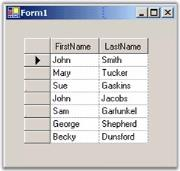# Binding to an Array list in Windows Forms GridDataBoundGrid(Classic)

26 Apr 20214 minutes to read

You can bind an ArrayList that holds objects with public properties. Given below is an example, which substantiates this point.

1. We first define an object called Person with two public properties: FirstName and LastName.
2. We then create an ArrayList holding a collection of these objects.
3. To bind this ArrayList to Grid Data Bound Grid, we set the grid’s DataSource property after dropping Grid Data Bound Grid onto the form.

Given below is the code sample for this.

``````//Creates the Person object class.

public class Person
{
private string lastName;
private string firstName;

public Person(string firstName, string lastName)
{
this.firstName = firstName;
this.lastName = lastName;
}

public string FirstName
{
get{return firstName;}
set{firstName = value;}
}

public string LastName
{
get{return lastName;}
set{lastName = value;}
}
}

private void Form1_Load(object sender, System.EventArgs e)
{
ArrayList al = new ArrayList();
this.gridDataBoundGrid1.DataSource = al;
}``````
``````'Creates the Person object class.

Public Class Person
Private firstName As String
Private lastName As String

Public Sub New(ByVal firstName As String, ByVal lastName As String)
Me.firstName = firstName
Me.lastName = lastName
End Sub

Public Property LastName()
Get
Return lastName
End Get
Set(ByVal Value)
lastName = Value
End Set
End Property

Public Property FirstName()
Get
Return firstName
End Get
Set(ByVal Value)
firstName = Value
End Set
End Property
End Class

Private Sub Form1_Load(ByVal sender As System.Object, ByVal e As System.EventArgs) Handles MyBase.Load
Dim al As ArrayList = New ArrayList()
Me.GridDataBoundGrid1.DataSource = al
End Sub``````

NOTE